# An Overview of the Logistic Regression and Regularization

Cengiz Zopluoglu (University of Oregon)
11/3/2022

[Updated: Sun, Nov 27, 2022 - 14:05:58 ]

# 1. Overview of the Logistic Regression

Logistic regression is a type of model that can be used to predict a binary outcome variable. Linear and logistic regression are indeed members of the same family of models called generalized linear models. While linear regression can also technically be used to predict a binary outcome, the bounded nature of a binary outcome, [0,1], makes the linear regression solution suboptimal. Logistic regression is a more appropriate model that considers the bounded nature of the binary outcome when making predictions.

The binary outcomes can be coded in various ways in the data, such as 0 vs. 1, True vs. False, Yes vs. No, and Success vs. Failure. The rest of the notes assume that the outcome variable is coded as 0s and 1s. We are interested in predicting the probability that future observation will belong to the class of 1s. The notes in this section will first introduce a suboptimal solution to predict a binary outcome by fitting a linear probability model using linear regression and discuss the limitations of this approach. Then, the logistic regression model and its estimation will be demonstrated. Finally, we will discuss various metrics that can be used to evaluate the performance of a logistic regression model.

Throughout these notes, we will use the Recidivism dataset from the NIJ competition to discuss different aspects of logistic regression and demonstrations. This data and variables in this data were discussed in detail in Lecture 1 and Lecture 2a – Section 6. The outcome of interest to predict in this dataset is whether or not an individual will be recidivated in the second year after initial release. To make demonstrations easier, I randomly sample 20 observations from this data. Eight observations in this data have a value of 1 for the outcome (recidivated), and 12 observations have a value of 0 (not recidivated).

# Import the randomly sample 20 observations from the recidivism dataset

# Outcome variable

table(recidivism_sub$Recidivism_Arrest_Year2)  0 1 12 8  ## 1.1. Linear Probability Model A linear probability model fits a typical regression model to a binary outcome. When the outcome is binary, the predictions from a linear regression model can be considered as the probability of the outcome being equal to 1, $\hat{Y} = P(Y = 1).$ Suppose we want to predict the recidivism in the second year (Recidivism_Arrest_Year2) by using the number of dependents they have. Then, we could fit this using the lm function. $\hat{Y} = P(Y = 1) = \beta_0 + \beta_1X + \epsilon$ mod <- lm(Recidivism_Arrest_Year2 ~ 1 + Dependents, data = recidivism_sub) summary(mod)  Call: lm(formula = Recidivism_Arrest_Year2 ~ 1 + Dependents, data = recidivism_sub) Residuals: Min 1Q Median 3Q Max -0.7500 -0.0625 0.0000 0.2500 0.5000 Coefficients: Estimate Std. Error t value Pr(>|t|) (Intercept) 0.75000 0.12949 5.792 0.0000173 *** Dependents -0.25000 0.06825 -3.663 0.00178 ** --- Signif. codes: 0 '***' 0.001 '**' 0.01 '*' 0.05 '.' 0.1 ' ' 1 Residual standard error: 0.3909 on 18 degrees of freedom Multiple R-squared: 0.4271, Adjusted R-squared: 0.3953 F-statistic: 13.42 on 1 and 18 DF, p-value: 0.001779 The intercept is 0.75 and the slope for the predictor, Dependents, is -.25. We can interpret the intercept and slope as the following for this example. Note that the predicted values from this model can now be interpreted as probability predictions because the outcome is binary. • Intercept (0.75): When the number of dependents is equal to 0, the probability of being recidivated in Year 2 is 0.75. • Slope (-0.25): For every additional dependent (one unit increase in X) the individual has, the probability of being recidivated in Year 2 is reduced by .25. The intercept and slope still represent the best-fitting line to our data, and this fitted line can be shown here.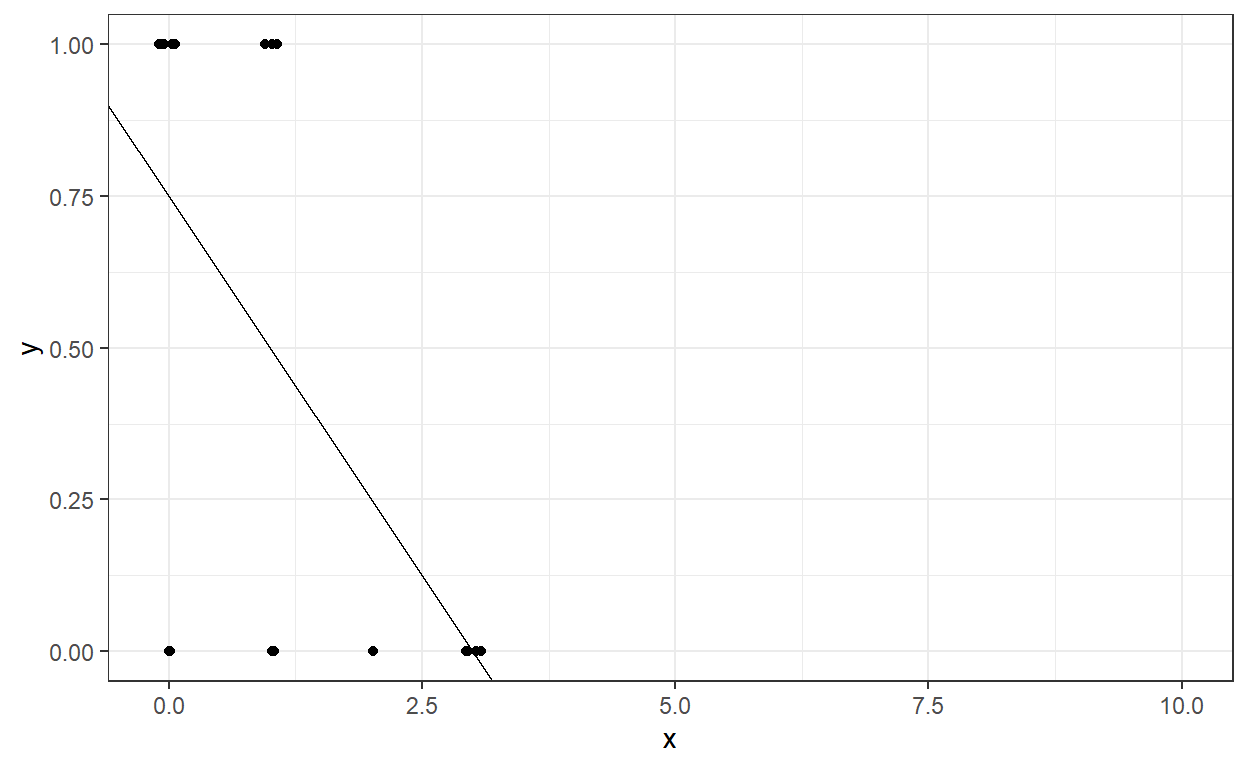Suppose we want to calculate the model’s predicted probability of being recidivated in Year 2 for a different number of dependents a parolee has. Let’s assume that the number of dependents can be from 0 to 10. What would be the predicted probability of being recidivated in Year 2 for a parolee with eight dependents? X <- data.frame(Dependents = 0:10) predict(mod,newdata = X)  1 2 0.7500000000000000000000 0.4999999999999999444888 3 4 0.2499999999999998889777 -0.0000000000000002220446 5 6 -0.2500000000000002220446 -0.5000000000000002220446 7 8 -0.7500000000000004440892 -1.0000000000000004440892 9 10 -1.2500000000000004440892 -1.5000000000000004440892 11 -1.7500000000000004440892  It is not reasonable for a probability prediction to be negative. One of the major issues with using linear regression to predict a binary outcome using a linear-probability model is that the model predictions can go outside of the boundary [0,1] and yield unreasonable predictions. So, a linear regression model may not be the best tool to predict a binary outcome. We should use a model that respects the boundaries of the outcome variable. ## 1.2. Description of Logistic Regression Model To overcome the limitations of the linear probability model, we bundle our prediction model in a sigmoid function. Suppose there is a real-valued function of $$a$$ such that $f(a) = \frac{e^a}{1 + e^a}.$ The output of this function is always between 0 and 1 regardless of the value of $$a$$. The sigmoid function is an appropriate choice for the logistic regression because it assures that the output is always bounded between 0 and 1. Note that this function can also be written as the following, and they are mathematically equivalent. $f(a) = \frac{1}{1 + e^{-a}}.$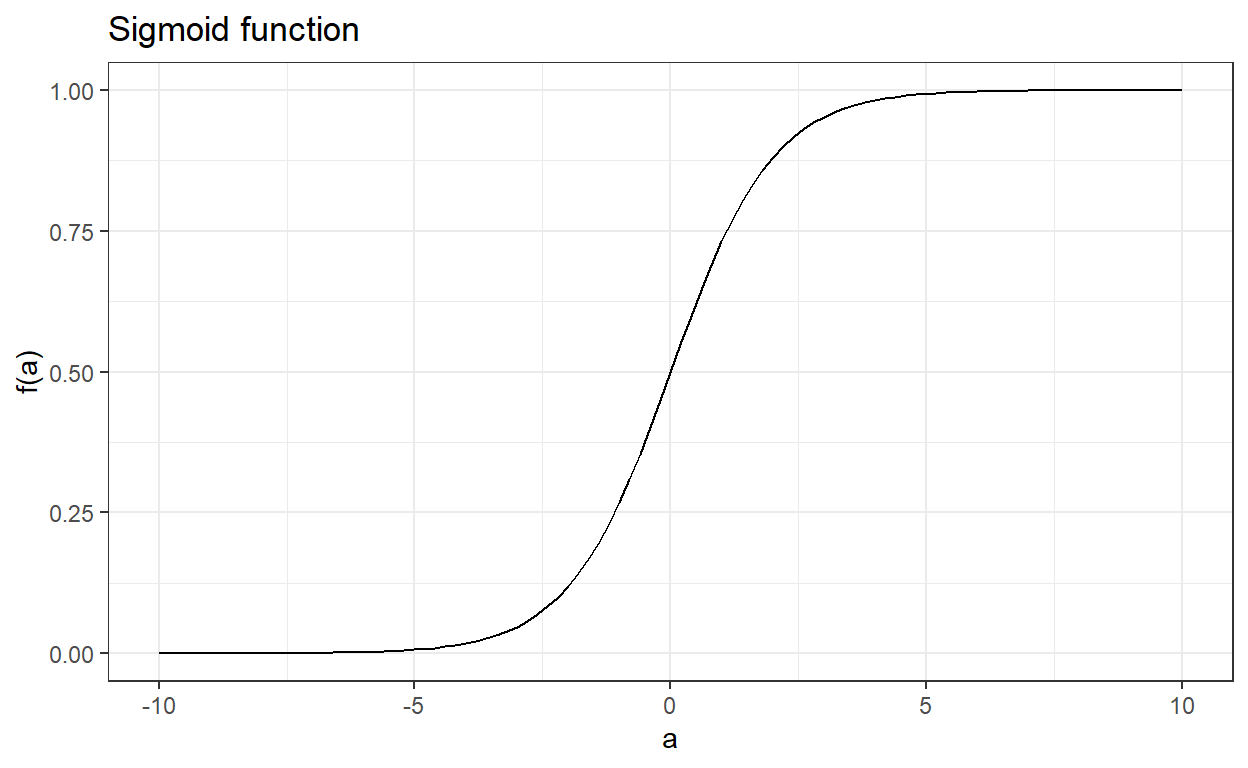If we revisit the previous example, we can specify a logistic regression model to predict the probability of being recidivated in Year 2 as the following by using the number of dependents a parolee has as the predictor, $P(Y=1) = \frac{1}{1 + e^{-(\beta_0+\beta_1X)}}.$ When values of a predictor variable is entered into the equation, the model output can be directly interpreted as the probability of the binary outcome being equal to 1 (or whatever category and meaning a value of 1 represents). Then, we assume that the actual outcome follows a binomial distribution with the predicted probability. $P(Y=1) = p$ $Y \sim Binomial(p)$ Suppose the coefficient estimates of this model are $$\beta_0$$=1.33 and $$\beta_1$$=-1.62. Then, for instance, we can compute the probability of being recidivated for a parolee with 8 dependents as the following: $P(Y=1) = \frac{1}{1+e^{-(1.33-1.62 \times 8)}} = 0.0000088951098.$ b0 = 1.33 b1 = -1.62 x = 0:10 y = 1/(1+exp(-(b0+b1*x))) data.frame(number.of.dependents = x, probability=y)  number.of.dependents probability 1 0 0.7908406347869 2 1 0.4280038670685 3 2 0.1289808521462 4 3 0.0284705876901 5 4 0.0057659655589 6 5 0.0011463789579 7 6 0.0002270757033 8 7 0.0000449461727 9 8 0.0000088951098 10 9 0.0000017603432 11 10 0.0000003483701In its original form, it is difficult to interpret the logistic regression parameters because a one unit increase in the predictor is no longer linearly related to the probability of the outcome being equal to 1 due to the nonlinear nature of the sigmoid function. The most common presentation of logistic regression is obtained after a bit of algebraic manipulation to rewrite the model equation. The logistic regression model above can also be specified as the following without any loss of meaning as they are mathematically equivalent. $ln \left [ \frac{P(Y=1)}{1-P(Y=1)} \right] = \beta_0+\beta_1X.$ The term on the left side of the equation is known as the logit. It is essentially the natural logarithm of odds. So, when the outcome is a binary variable, the logit transformation of the probability that the outcome is equal to 1 can be represented as a linear equation. It provides a more straightforward interpretation. For instance, we can now say that when the number of dependents is equal to zero, the predicted logit is equal to 1.33 (intercept), and for every additional dependent, the logit decreases by 1.62 (slope). It is common to transform the logit to odds when interpreting the parameters. For instance, we can say that when the number of dependents is equal to zero, the odds of being recidivated is 3.78 ($$e^{1.33}$$), and for every additional dependent the odds of being recidivated is reduced by about 80% ($$1 - e^{-1.62}$$). The right side of the equation can be expanded by adding more predictors, adding polynomial terms of the predictors, or adding interactions among predictors. A model with only the main effects of $$P$$ predictors can be written as $ln \left [ \frac{P(Y=1)}{1-P(Y=1)} \right] = \beta_0 + \sum_{p=1}^{P} \beta_pX_{p},$ and the coefficients can be interpreted as • $$\beta_0$$: the predicted logit when the values for all the predictor variables in the model are equal to zero. $$e^{\beta_0}$$, the predicted odds of the outcome being equal to 1 when the values for all the predictor variables in the model are equal to zero. • $$\beta_p$$: the change in the predicted logit for one unit increases in $$X_p$$ when the values for all other predictors in the model are held constant. For every one unit in increase in $$X_p$$, the odds of the outcome being equal to 1 is multiplied by $$e^{\beta_p}$$ when the values for all other predictors in the model are held constant. In other words, $$e^{\beta_p}$$ is odds ratio, the ratio of odds at $$\beta_p = a+1$$ to the odds at $$\beta_p = a$$. It is essential that you get familiar with the three concepts (probability, odds, logit) and how these three are related to each other for interpreting the logistic regression parameters.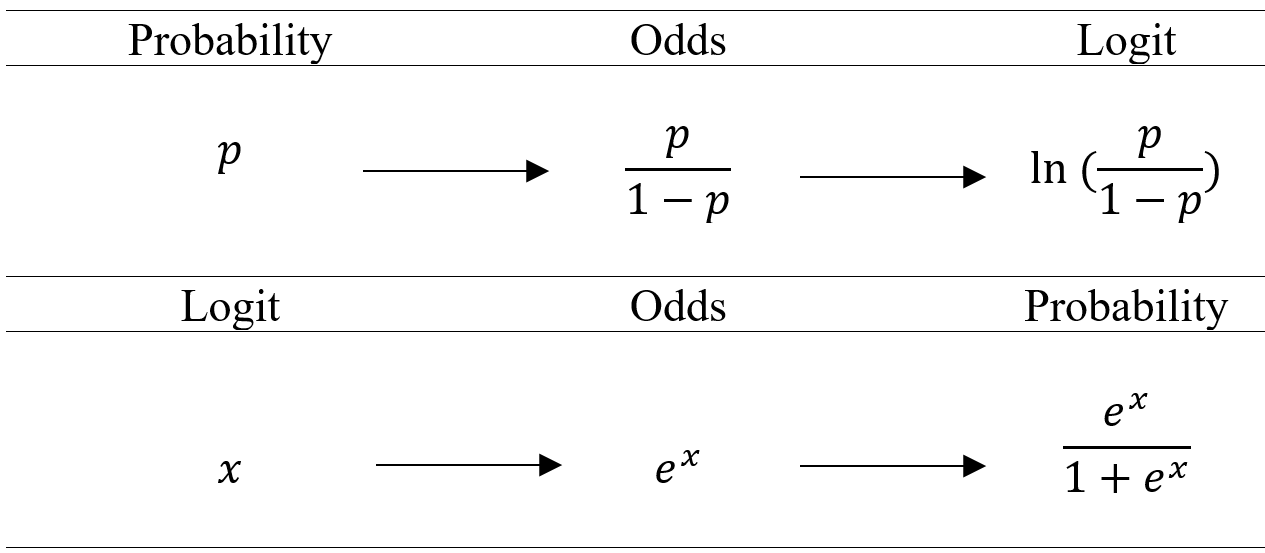NOTE The sigmoid function is not the only tool to be used for modeling a binary outcome. One can also use the cumulative standard normal distribution function, $$\phi(a)$$, and the output of $$\phi(a)$$ is also bounded between 0 and 1. When $$\phi$$ is used to transform the prediction model, this is known as probit regression and serves the same purpose as the logistic regression, which is to predict the probability of a binary outcome being equal to 1. However, it is always easier and more pleasant to work with logarithmic functions, which have better computational properties. Therefore, logistic regression is more commonly used than probit regression. ## 1.3. Model Estimation ### 1.3.1. The concept of likelihood It is essential to understand the likelihood concept for estimating the coefficients of a logistic regression model. We will consider a simple example of flipping coins for this. Suppose you flip the same coin 20 times and observe the following outcome. We don’t know whether this is a fair coin in which the probability of observing a head or tail is equal to 0.5. $\mathbf{Y} = \left ( H,H,H,T,H,H,H,T,H,T \right )$ Suppose we define $$p$$ as the probability of observing a head when we flip this coin. By definition, the probability of observing a tail is $$1-p$$. $P(Y=H) = p$ $P(Y=T) = 1 - p$ Then, we can calculate the likelihood of our observations of heads and tails as a function of $$p$$. $\mathfrak{L}(\mathbf{Y}|p) = p \times p \times p \times (1-p) \times p \times p \times p \times (1-p) \times p \times (1-p)$ $\mathfrak{L}(\mathbf{Y}|p) = p^7 \times (1-p)^3$ For instance, if we say that this is a fair coin and, therefore, $$p$$ is equal to 0.5, then the likelihood of observing seven heads and three tails would be equal to $\mathfrak{L}(\mathbf{Y}|p = 0.5) = 0.5^7 \times (1-0.5)^3 = 0.0009765625$ On the other hand, another person can say this is probably not a fair coin, and the $$p$$ should be something higher than 0.5. How about 0.65? $\mathfrak{L}(\mathbf{Y}|p = 0.65) = 0.65^7 \times (1-0.65)^3 = 0.00210183$ Based on our observation, we can say that an estimate of $$p$$ being equal to 0.65 is more likely than an estimate of $$p$$ being equal to 0.5. Our observation of 7 heads and 3 tails is more likely if we estimate $$p$$ as 0.65 rather than 0.5. ### 1.3.2. Maximum likelihood estimation (MLE) Then, what would be the best estimate of $$p$$ given our observed data (seven heads and three tails)? We can try every possible value of $$p$$ between 0 and 1 and calculate the likelihood of our data ($$\mathbf{Y}$$). Then, we can pick the value that makes our data most likely (largest likelihood) to observe as our best estimate. Given the data we observed (7 heads and three tails), it is called the maximum likelihood estimate of $$p$$. p <- seq(0,1,.001) L <- p^7*(1-p)^3 ggplot()+ geom_line(aes(x=p,y=L)) + theme_bw() + xlab('Probability of Observing a Head (p)')+ ylab('Likelihood of Observing 7 Heads and 3 Tails')+ geom_vline(xintercept=p[which.max(L)],lty=2)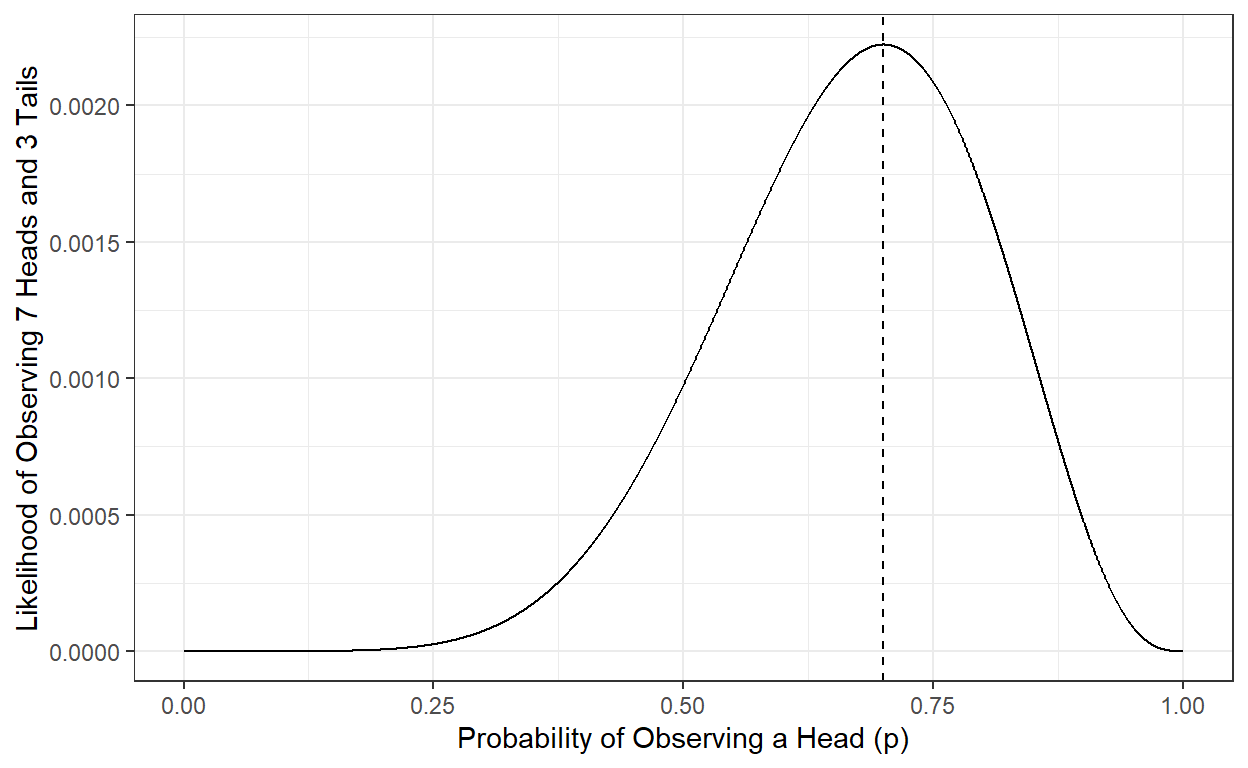We can show that the $$p$$ value that makes the likelihood largest is 0.7, and the likelihood of observing seven heads and three tails is 0.002223566 when $$p$$ is equal to 0.7. Therefore, the maximum likelihood estimate of the probability of observing a head for this particular coin is 0.7, given the ten observations we have made. L[which.max(L)]  0.002223566 p[which.max(L)]  0.7 Note that our estimate can change and be updated if we continue collecting more data by flipping the same coin and recording our observations. ### 1.3.3. The concept of the log-likelihood The computation of likelihood requires the multiplication of so many $$p$$ values, and when you multiply values between 0 and 1, the result gets smaller and smaller. It creates problems when you multiply so many of these small $$p$$ values due to the maximum precision any computer can handle. For instance, you can see the minimum number that can be represented in R in your local machine. .Machine$double.xmin
 2.225074e-308

When you have hundreds of thousands of observations, it is probably not a good idea to work directly with likelihood. Instead, we work with the log of likelihood (log-likelihood). The log-likelihood has two main advantages:

• We are less concerned about the precision of small numbers our computer can handle.

• Log-likelihood has better mathematical properties for optimization problems (the log of the product of two numbers equals the sum of the log of the two numbers).

• The point that maximizes likelihood is the same number that maximizes the log-likelihood, so our end results (MLE estimate) do not care if we use log-likelihood instead of likelihood.

$ln(\mathfrak{L}(\mathbf{Y}|p)) = ln(lop^7 \times (1-p)^3)$ $ln(\mathfrak{L}(\mathbf{Y}|p)) = ln(p^7) + ln((1-p)^3)$ $ln(\mathfrak{L}(\mathbf{Y}|p)) = 7 \times ln(p) + 3 \times ln(1-p)$

p <- seq(0,1,.001)

logL <- log(p)*7 + log(1-p)*3

ggplot()+
geom_line(aes(x=p,y=logL)) +
theme_bw() +
xlab('Probability of Observing a Head (p)')+
ylab('Loglikelihood of Observing 7 Heads and 3 Tails')+
geom_vline(xintercept=p[which.max(logL)],lty=2)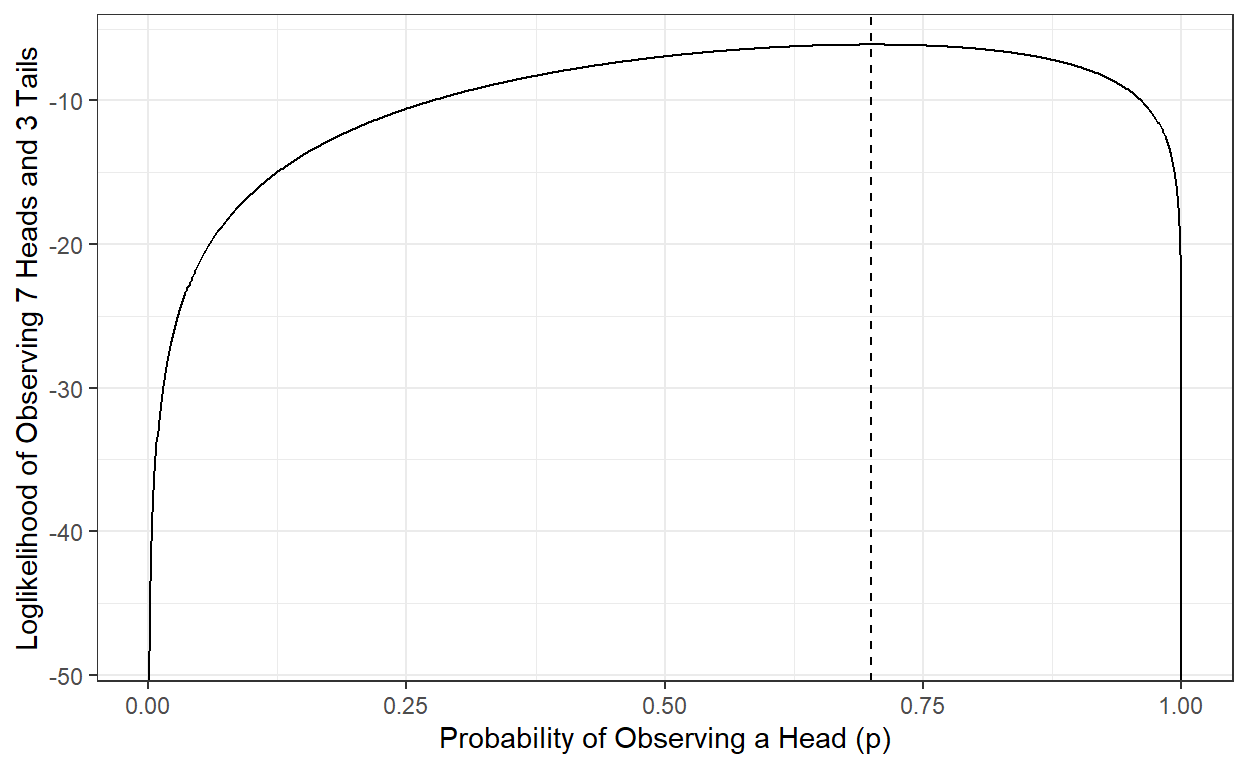logL[which.max(logL)]
 -6.108643
p[which.max(logL)]
 0.7

### 1.3.4. MLE for Logistic Regression coefficients

Now, we can apply these concepts to estimate the logistic regression coefficients. Let’s revisit our previous example in which we predict the probability of being recidivated in Year 2, given the number of dependents a parolee has. Our model can be written as the following.

$ln \left [ \frac{P_i(Y=1)}{1-P_i(Y=1)} \right] = \beta_0+\beta_1X_i.$

Note that $$X$$ and $$P$$ have a subscript $$i$$ to indicate that each individual may have a different X value, and therefore each individual will have a different probability. Our observed outcome is a set of 0s and 1s. Remember that eight individuals were recidivated (Y=1), and 12 were not recidivated (Y=0).

recidivism_sub$Recidivism_Arrest_Year2   1 1 0 0 1 1 1 1 0 0 0 0 1 0 0 1 0 0 0 0 Given a set of coefficients, {$$\beta_0,\beta_1$$}, we can calculate the logit for every observation using the model equation and then transform this logit to a probability, $$P_i(Y=1)$$. Finally, we can calculate the log of the probability for each observation and sum them across observations to obtain the log-likelihood of observing this set of observations (12 zeros and eight ones). Suppose that we have two guesstimates for {$$\beta_0,\beta_1$$}, which are 0.5 and -0.8, respectively. These coefficients imply the following predicted model.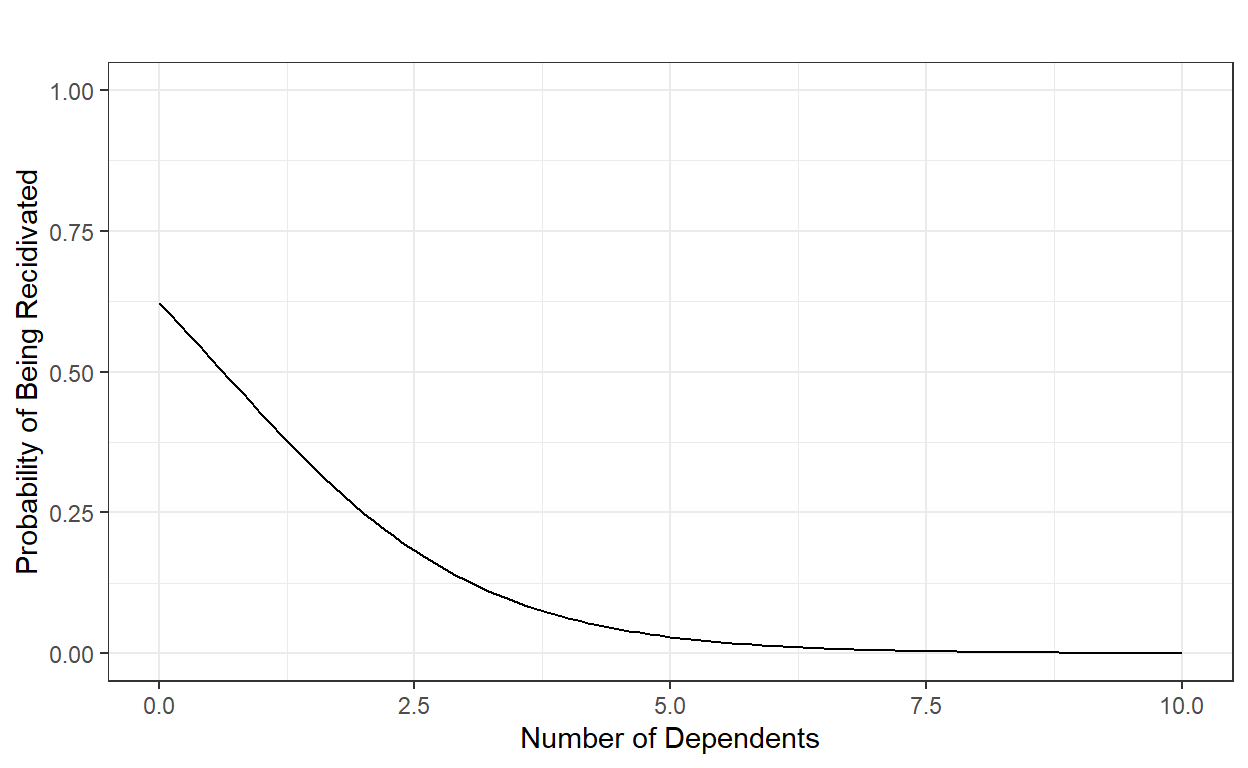If these two coefficients were our estimates, how likely would we observe the outcome in our data, given the number of dependents? The below R code first finds the predicted logit for every observation, assuming that $$\beta_0$$ = 0.5 and $$\beta_1$$ = -0.8. b0 = 0.5 b1 = -0.8 x = recidivism_sub$Dependents

y = recidivism_sub$Recidivism_Arrest_Year2 pred_logit <- b0 + b1*x pred_prob1 <- exp(pred_logit)/(1+exp(pred_logit)) pred_prob0 <- 1 - pred_prob1 data.frame(Dependents = x, Recidivated = y, Prob1 = pred_prob1, Prob0 = pred_prob0)  Dependents Recidivated Prob1 Prob0 1 0 1 0.6224593 0.3775407 2 1 1 0.4255575 0.5744425 3 2 0 0.2497399 0.7502601 4 1 0 0.4255575 0.5744425 5 1 1 0.4255575 0.5744425 6 0 1 0.6224593 0.3775407 7 0 1 0.6224593 0.3775407 8 1 1 0.4255575 0.5744425 9 3 0 0.1301085 0.8698915 10 0 0 0.6224593 0.3775407 11 1 0 0.4255575 0.5744425 12 0 0 0.6224593 0.3775407 13 0 1 0.6224593 0.3775407 14 3 0 0.1301085 0.8698915 15 3 0 0.1301085 0.8698915 16 0 1 0.6224593 0.3775407 17 3 0 0.1301085 0.8698915 18 3 0 0.1301085 0.8698915 19 3 0 0.1301085 0.8698915 20 3 0 0.1301085 0.8698915 logL <- y*log(pred_prob1) + (1-y)*log(pred_prob0) sum(logL)  -9.253358 We can summarize this by saying that if our model coefficients were $$\beta_0$$ = 0.5 and $$\beta_1$$ = -0.8, then the log of the likelihood of observing the outcome in our data would be -9.25. $\mathbf{Y} = \left ( 1,0,1,0,0,0,0,1,1,0,0,1,0,0,0,1,0,0,0,0 \right )$ $\mathfrak{logL}(\mathbf{Y}|\beta_0 = 0.5,\beta_1 = -0.8) = -9.25$ The critical question is whether or not there is another pair of values we can assign to $$\beta_0$$ and $$\beta_1$$ that would provide a higher likelihood of data. If there are, then they would be better estimates for our model. If we can find such a pair with the maximum log-likelihood of data, then they would be our maximum likelihood estimates for the given model. We can approach this problem crudely to gain some intuition about Maximum Likelihood Estimation. Suppose that a reasonable range of values for $$\beta_0$$ is from -3 to 3, and for $$\beta_1$$ is from -3 to 3. Let’s think about every possible combinations of values for $$\beta_0$$ and $$\beta_1$$ within these ranges with increments of .01. Then, let’s calculate the log-likelihood of data for every possible combination and plot these in a 3D plot as a function of $$\beta_0$$ and $$\beta_1$$. grid <- expand.grid(b0=seq(-3,3,.01),b1=seq(-3,3,.01)) grid$logL <- NA

for(i in 1○:nrow(grid)){

x = recidivism_sub$Dependents y = recidivism_sub$Recidivism_Arrest_Year2

pred_logit    <- grid[i,]$b0 + grid[i,]$b1*x
pred_prob1    <- exp(pred_logit)/(1+exp(pred_logit))
pred_prob0    <- 1 - pred_prob1
logL          <- y*log(pred_prob1) + (1-y)*log(pred_prob0)
grid[i,]$logL <- sum(logL) print(i) } require(plotly) plot_ly(grid, x = ~b0, y = ~b1, z = ~logL, marker = list(color = ~logL, showscale = FALSE, cmin=min(grid$logL),
cmax=max(grid\$logL),cauto=F),
width=600,height=600) %>%
add_markers()